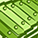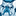# QlikView App Dev

Discussion Board for collaboration related to QlikView App Development.

Announcements
QlikWorld 2023, a live, in-person thrill ride. Save \$300 before February 6: REGISTER NOW!
cancel
Showing results for
Did you mean:Creator II

## Null Count

Hi Experts,

I am trying to calculate the Null values present in Value field. Here the nullcount under Value is 17.

Please provide the solution to calculate the Nullcount under value field apart from below two methods.

=Count( IF(ISNULL(Value) OR LEN(trim(Value))=0 OR Value=0,1))

=NullCount(Value)

 ID Value 1 -1301 2 3 4 7 5 6 63 7 8 77 9 10 11 12 13 14 15 16 71 17 18 19 20 32 21 22 45 23 24 38 25 26 41

1 Solution

Accepted SolutionsChampion III

May use a NullAsValue and count on that Field. Like

NULLASVALUE Value;

SET NullValue = '<NoData>';

FROM yoursource;

Then use like

= Count({< Value = {'<NoData>'} >} ID )

14 RepliesMVP

May be this

Sum(-Len(Trim(Value)) = 0)MVP

My bad, this is the right syntax

=-Sum(Len(Trim(Value)) = 0)MVP

Or this?

=Count({<Value -= {"=Len(Value)>0"}>} Value)

Before develop something, think If placed (The Right information | To the right people | At the Right time | In the Right place | With the Right context)MVP

If Value is truly null, then this won't work.... but this can

=Count({<ID = {"=Len(Value) = 0"}>} Value)MVP

Not working? Which one is not working?Creator II
Author

=Count({<ID = {"=Len(Value) = 0"}>} Value)

This expression is not working getting 0 instead of 17MVP

=Count({<ID = {"=Len(Value) = 0"}>} ID)Specialist

Hi,

Try

=Count(if(IsNull(Value)or Value='',1))

Regards.Master II

One more way:

If you are sure the Value field is always a number then the following will work:

=Count(If(Not IsNum(Value),1))Community Browser